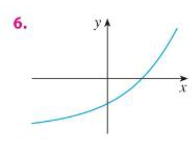Chapter 1.5, Problem 6E### Single Variable Calculus: Early Tr...

8th Edition
James Stewart
ISBN: 9781305270343

#### Solutions

Chapter
Section### Single Variable Calculus: Early Tr...

8th Edition
James Stewart
ISBN: 9781305270343
Textbook Problem

# A function is given by a table of values, a graph, a formula or a verbal description. Determine whether it is one-to-one.To determine

Whether the function given by a graph is one-to-one.

Explanation

Result used:

Horizontal line test states that the graph of the function is one-to-one function if and only if a horizontal line intersects the graph exactly once.

Calculation:

Perform the horizontal line test for the given graph.

Draw a horizontal line in such a way that it passes through the curve as shown in Figure 1...

### Still sussing out bartleby?

Check out a sample textbook solution.

See a sample solution

#### The Solution to Your Study Problems

Bartleby provides explanations to thousands of textbook problems written by our experts, many with advanced degrees!

Get Started

#### Proof prove that cos(sin1x)=1x2

Calculus: Early Transcendental Functions

#### In Exercises 7-12, solve for y in terms of x. x=2y14

Calculus: An Applied Approach (MindTap Course List)

#### Find for x = 3t2 + 1, y = t6 + 6t5. t4 + 5t3 4t3 + 15t2

Study Guide for Stewart's Multivariable Calculus, 8th